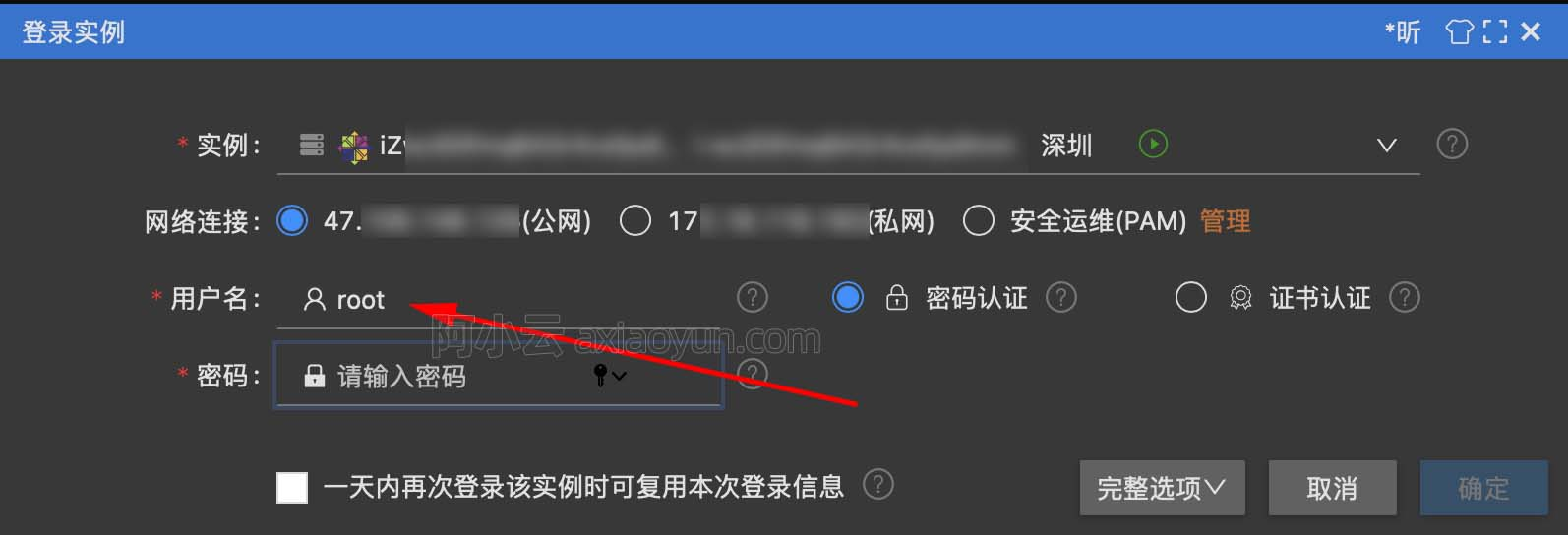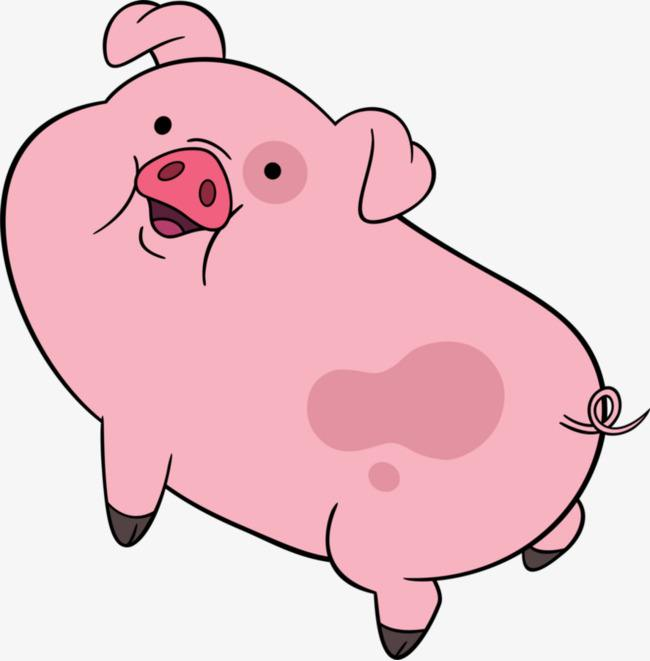[kata] Playing with digits

+关注继续查看
package kata_011;

/**
* Some numbers have funny properties. For example:
*
* 89 --> 8¹ + 9² = 89 * 1
*
* 695 --> 6² + 9³ + 5⁴= 1390 = 695 * 2
*
* 46288 --> 4³ + 6⁴+ 2⁵ + 8⁶ + 8⁷ = 2360688 = 46288 * 51
*
* Given a positive integer n written as abcd... (a, b, c, d... being digits)
* and a positive integer p we want to find a positive integer k, if it exists,
* such as the sum of the digits of n taken to the successive powers of p is
* equal to k * n. In other words:
*
* Is there an integer k such as : (a ^ p + b ^ (p+1) + c ^(p+2) + d ^ (p+3) + ...) = n * k
*
* If it is the case we will return k, if not return -1.
*
* Note: n, p will always be given as strictly positive integers.
*
* digPow(89, 1) should return 1 since 8¹ + 9² = 89 = 89 * 1 digPow(92, 1)
* should return -1 since there is no k such as 9¹ + 2² equals 92 * k
* digPow(695, 2) should return 2 since 6² + 9³ + 5⁴= 1390 = 695 * 2
* digPow(46288, 3) should return 51 since 4³ + 6⁴+ 2⁵ + 8⁶ + 8⁷ = 2360688 = 46288 * 51
*
* @author SeeClanUkyo
*
*/
public class DigPow {
public static void main(String[] args) {

System.out.println(digPow(46288, 3));
}

public static long digPow(int n, int p) {
if (n > 0) {
String nstr = n + "";
int nlen = nstr.length();

long sum = 0;
for (int i = 0; i < nlen; i++) {
sum += Math.pow(Integer.parseInt(nstr.substring(i, i + 1)), (p + i));
if (sum % n == 0) {
return sum / n;
}
}

}
return -1;
}
}9963 06726 014450 013719 0windows server 2008阿里云ECS服务器安全设置

9134 07453 07315 054708 022342 04442 0
118

0

《2021云上架构与运维峰会演讲合集》

《零基础CSS入门教程》

《零基础HTML入门教程》